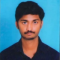## Divide:(i) $x+2x^2+3x^4-x^5$ by $2x$(ii) $y^4-3y^3+\frac{1}{2y^2}$ by $3y$(iii) $-4a^3+4a^2+a$ by $2a$Akhileshwar Nani

Updated on 13-Apr-2023 22:54:39

37 Views

Given:The given expressions are:(i) $x+2x^2+3x^4-x^5$ by $2x$(ii) $y^4-3y^3+\frac{1}{2y^2}$ by $3y$(iii) $-4a^3+4a^2+a$ by $2a$To do:We have to divide the given expressions.Solution:We have to divide the given polynomials by monomials using the formula $x^a \div x^b=a^{a-b}$Polynomials: Polynomials are expressions in which each term is a constant multiplied by a variable raised to a ... Read More

## Simplify:(i) $\frac{16m^3y^2}{4m^2y}$(ii) $\frac{32m^2n^3p^2}{4mnp}$Akhileshwar Nani

Updated on 13-Apr-2023 22:53:11

23 Views

Given:The given expressions are:(i) $\frac{16m^3y^2}{4m^2y}$(ii) $\frac{32m^2n^3p^2}{4mnp}$To do:We have to simplify the given expressions.Solution:We have to divide the given polynomials by monomials using the formula $x^a \div x^b=a^{a-b}$Polynomials: Polynomials are expressions in which each term is a constant multiplied by a variable raised to a whole number power.Monomial:A monomial is an expression ... Read More

## Divide:(i) $-21abc^2$ by $7abc$(ii) $72xyz^2$ by $-9xz$(iii) $-72a^4b^5c^8$ by $-9a^2b^2c^3$Akhileshwar Nani

Updated on 13-Apr-2023 22:52:27

42 Views

Given:The given expressions are:(i) $-21abc^2$ by $7abc$(ii) $72xyz^2$ by $-9xz$(iii) $-72a^4b^5c^8$ by $-9a^2b^2c^3$To do:We have to divide the given expressions.Solution:We have to divide the given polynomials by monomials using the formula $x^a \div x^b=a^{a-b}$Polynomials: Polynomials are expressions in which each term is a constant multiplied by a variable raised to ... Read More

## Divide:(i) $6x^3y^2z^2$ by $3x^2yz$(ii) $15m^2n^3$ by $5m^2n^2$(iii) $24a^3b^3$ by $-8ab$Akhileshwar Nani

Updated on 13-Apr-2023 22:51:38

116 Views

Given:The given expressions are:(i) $6x^3y^2z^2$ by $3x^2yz$(ii) $15m^2n^3$ by $5m^2n^2$(iii) $24a^3b^3$ by $-8ab$To do:We have to divide the given expressions.Solution:We have to divide the given polynomials by monomials using the formula $x^a \div x^b=a^{a-b}$Polynomials: Polynomials are expressions in which each term is a constant multiplied by a variable raised to a ... Read More

## Write each of the following polynomials in the standard form. Also, write their degree.(i) $x^2+3+6x+5x^4$(ii) $a^2+4+5a^6$(iii) $(x^3-1)(x^3-4)$(iv) $(a^3-\frac{3}{8})(a^3+\frac{16}{17})$(v) $(a+\frac{3}{4})(a+\frac{4}{3})$Akhileshwar Nani

Updated on 13-Apr-2023 22:50:51

31 Views

Given:The given expressions are:(i) $x^2+3+6x+5x^4$(ii) $a^2+4+5a^6$(iii) $(x^3-1)(x^3-4)$(iv) $(a^3-\frac{3}{8})(a^3+\frac{16}{17})$(v) $(a+\frac{3}{4})(a+\frac{4}{3})$To do:We have to write the standard form of the given polynomials and find their degree.Solution:Polynomials: Polynomials are expressions in which each term is a constant multiplied by a variable raised to a whole number power.The standard form of the polynomial is a polynomial ... Read More

## Which of the following expressions are not polynomials?:(i) $x^2+2x^{-2}$(ii) $\sqrt{ax}+x^2-x^3$(iii) $3y^3-\sqrt{5}y+9$(iv) $ax^{\frac{1}{2}}y^7+ax+9x^2+4$(v) $3x^{-3}+2x^{-1}+4x+5$Akhileshwar Nani

Updated on 13-Apr-2023 22:49:54

73 Views

Given:The given expressions are:(i) $x^2+2x^{-2}$(ii) $\sqrt{ax}+x^2-x^3$(iii) $3y^3-\sqrt{5}y+9$(iv) $ax^{\frac{1}{2}}y^7+ax+9x^2+4$(v) $3x^{-3}+2x^{-1}+4x+5$To do:We have to find which of the given expressions are polynomials.Solution:Polynomials: Polynomials are expressions in which each term is a constant multiplied by a variable raised to a whole number power.To identify whether the given expression is polynomial, check if all the ... Read More

## Write the degree of each of the following polynomials:(i) $2x^3+5x^2-7$(ii) $5x^2-3x+2$(iii) $2x+x^2-8$(iv) $\frac{1}{2}y^7-12y^6+48y^5-10$(v) $3x^3+1$(vi) $5$(vii) $20x^3+12x^2y^2-10y^2+20$Akhileshwar Nani

Updated on 12-Apr-2023 19:48:15

153 Views

Given:The given polynomials are:(i) $2x^3+5x^2-7$(ii) $5x^2-3x+2$(iii) $2x+x^2-8$(iv) $\frac{1}{2}y^7-12y^6+48y^5-10$(v) $3x^3+1$(vi) $5$(vii) $20x^3+12x^2y^2-10y^2+20$To do:We have to find the degree of each of the given polynomials.Solution:Degree of a polynomial:The degree of a polynomial is the highest or the greatest power of a variable in the polynomial expression.To find the degree, identify the exponents ... Read More

## Factorize each of the following quadratic polynomials by using the method of completing the square:(i) $y^2-7y+12$(ii) $z^2-4z-12$Akhileshwar Nani

Updated on 12-Apr-2023 19:46:14

47 Views

Given:The given quadratic polynomials are:(i) $y^2-7y+12$(ii) $z^2-4z-12$To do:We have to factorize the given quadratic polynomials.Solution:Factorizing algebraic expressions:Factorizing an algebraic expression implies writing the expression as a product of two or more factors. Factorization is the reverse of distribution. An algebraic expression is factored completely when it is written as a product of ... Read More

## Factorize each of the following quadratic polynomials by using the method of completing the square:(i) $a^2+2a-3$(ii) $4x^2-12x+5$Akhileshwar Nani

Updated on 12-Apr-2023 19:44:47

45 Views

Given:The given quadratic polynomials are:(i) $a^2+2a-3$(ii) $4x^2-12x+5$To do:We have to factorize the given quadratic polynomials.Solution:Factorizing algebraic expressions:Factorizing an algebraic expression implies writing the expression as a product of two or more factors. Factorization is the reverse of distribution. An algebraic expression is factored completely when it is written as a product of ... Read More

## Factorize each of the following quadratic polynomials by using the method of completing the square:(i) $x^2+12x+20$(ii) $a^2-14a-51$Akhileshwar Nani

Updated on 12-Apr-2023 19:43:10

47 Views

Given:The given quadratic polynomials are:(i) $x^2+12x+20$(ii) $a^2-14a-51$To do:We have to factorize the given quadratic polynomials.Solution:Factorizing algebraic expressions:Factorizing an algebraic expression implies writing the expression as a product of two or more factors. Factorization is the reverse of distribution. An algebraic expression is factored completely when it is written as a product of ... Read More